## CALCULATION OF THE COEFFICIENT OF RELATIONSHIP

Common Ancestors Defined

Common ancestors are usually only shared by collateral relatives, since with direct relationships one individual is the ancestor of the other. Exceptions are shown here.

In its literal sense a 'common ancestor' is any person who passes genes directly to two or more individuals. However, for the purposes of calculating the coefficient of relationship (R) and the coefficient of inbreeding (F), it has a more restricted definition:-

In its narrow sense a common ancestor is any preceding individual in the pedigree who passes genes directly to two or more persons through different independent routes or paths. In the following diagram there are three individuals who satisfy this definition:- i.e. B, H and I, who are common ancestors of J and K. (Note that parents are common ancestors in this context).

Figure 7 Common Ancestors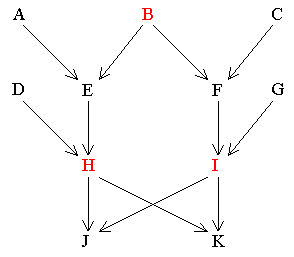B passes genes directly to J and K through the following independent routes:-

 (B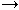EHJ) (BEHK) and also and (BFIK) (BFIJ)

Similarly H and I pass genes to J and K as follows:-

 (HJ) (IJ) and and (HK) (IK)

It can be seen that although A, C, D, E, F and G all pass genes directly to both J and K, they do not do so by different independent routes. e.g. The paths from A to J and K are:-

(AEHJ)

and

(AEHK)

They do not, therefore, qualify as common ancestors when calculating R . This distinction is important in finding all the relevant common ancestors in the next section. The contributions of A, C, D, E, F and G towards the degree of relationship between J and K are accounted for by the paths using H and I as common ancestors. The following section shows how to calculate the degree or coefficient of relationship.

The Coefficient of Relationship (R)

The standard procedure for measuring degrees of relationship quantitatively uses Sewall Wright's method of path coefficients (Wright, 1922). The measure, known as the coefficient of relationship (R), calculates the proportion of genes that two individuals have in common as a result of their genetic relationship. It is not an absolute measurement and is relative to the average relationship within the population at some reference point in the recent past (usually 3 to 5 generations back). Thus, some genes are common to all members of a closed population. For example, genes for white skin in native Europeans. Therefore, it is the proportion of genes held in common by two related individuals over and above those held in common by the whole population, since all animals of the same species are related to some extent.

The coefficient of relationship is not the same as the degree of resemblance (or correlation coefficient) between two relatives for the same character. In addition to R, the phenotypic correlation also depends on the heritability of the character. e.g. If the heritability of mature height is 0.4 in a certain population, then the average phenotypic correlation between full brothers, whose coefficient of relationship is 0.5, will be 0.4 x 0.5 = 0.2. For some traits the correlation between full sibs may be higher than indicated above because of a shared environment in early life and the effects of dominance interaction, (see here). For further treatment of this topic, Falconer (1989) is recommended.

The formula is as follows:

(RXY) = Σ (1/2)n 

Where RXYis the coefficient of relationship between the two relatives X and Y and n is the number of connecting links or paths separating them. Σ (meaning 'the sum of') refers to the fact that if there is more than one connecting path, the paths are computed separately and their coefficients are then added together. The type of relationship, i.e. whether direct or collateral, affects the procedure. The differences between direct and collateral relationship are explained fully in the next section.

Direct Relationship

The number of direct links between the individuals is counted and used in the above formula. An important rule with direct relationships is that the paths must always follow the direction of the arrows. The correct path in the following pedigree for RAI is:-ACEGI.

Figure 8 Direct Relationship Calculation** A table of values of (1/2)n is given here.

If there have been any cousin marriages there may be more than one path between ancestor and descendant. Thus, inbreeding increases genetic relationships above the standard values. An example is shown below:-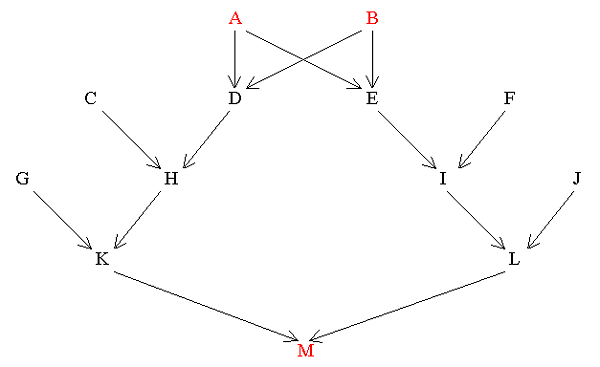Find RAM

 Paths (1/2)n A_1_D_2_H_3_K_4_M (1/2)4 = 1/16 A_1_E_2_ I _3_L_4_M (1/2)4 = 1/16 Σ(1/2)n = 1/8

Therefore RAM = 1/8 = 0.125 

Collateral Relationship

Two important rules must be observed for collaterals:-

a) The path must go back against the arrows from one collateral relative to the common ancestor, and then follow the arrows forward to the other collateral relative. For example, in the next pedigree diagram, one of the paths between G and H is:-G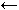DAEH.

b) No individual must occur in the same path more than once. In the pedigree diagram shown in Figure 11 the following path from I to J is not permissible because G occurs twice:- IGDGJ. The route from D to I must be independent of the route from D to J.

Figure 10 The Relationship between Single First Cousins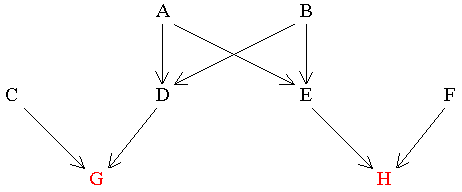In this case trace the path back from one cousin to the common ancestor and then forward to the other cousin. Remember, a qualifying common ancestor is someone who passes genes directly to both collateral relatives along different routes. With collateral relatives there is often more than one common ancestor.

Find RGH

 Common Ancestors  Paths (1/2)n A G_1_D_2_A_3_E_4_H (1/2)4 = 1/16 B G_1_D_2_B_3_E_4_H (1/2)4 = 1/16 Σ(1/2)n = 1/8

Therefore, RGH= 0.125

Cousin marriages will again increase the number of paths as they did with direct

relationships.

Figure 11 Two Brothers (I and J) whose Parents are First Cousins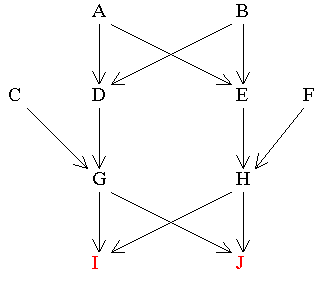Find RIJ

 Common Ancestors  Paths (1/2)n G I_1_G_2_J (1/2)2 = 1/4 H I_1_H_2_J (1/2)2 = 1/4 A I_1_G_2_D_3_A_4_E_5_H_6_J (1/2)6 1/64 I_1_H_2_E_3_A_4_D_5_G_6_J (1/2)6 1/64 B I_1_G_2_D_3_B_4_E_5_H_6_J (1/2)6 1/64 I_1_H_2_E_3_B_4_D_5_G_6_J (1/2)6 1/64 Σ(1/2)n 9/16

Therefore RIJ = 9/16 = 0.5625

If there had been no inbreeding the two brothers would only have a relationship of 0.5.

Direct and Collateral Relationships Combined (See here)

When one person is the ancestor of the other and they are also related collaterally because both are descended from a third person, it is usually more convenient to compute the direct relationship first. Then, after calculating the collateral relationship, the two measures are added together. In the following diagram M is the result of a marriage between two second cousins:

Figure 12 Combined Relationships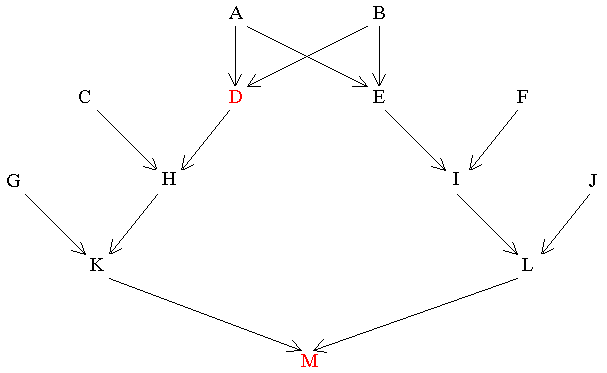Find RDM

 Common Ancestors Path (1/2)n Direct Relationship D_1_H_2_K_3_M (1/2)3 = 1/8 Collateral Relationship A D_1_A_2_E_3_I_4_L_5_M (1/2)5 = 1/32 B D_1_B_2_E_3_I_4_L_5_M (1/2)5 = 1/32 Σ (1/2)n = 3/16

Therefore, RDM = 3/16 = 0.1875

The Special Case of Identical Twins

Identical twins (also known as monozygotic twins) start life as a single fertilised egg, as opposed to fraternal (dizygotic) twins who originate from two fertile eggs. In the case of identical twins, the first cell division occurs normally, but the two resulting cells accidentally become detached and start to develop as separate individuals. Because they come from one original egg they are exact copies genetically. This means that they hold all their genes in common and have a genetic relationship of 1.0. How is this accommodated by the basic formula for the coefficient of relationship [R = ∑(1/2)n]?

The symbol n refers to the number of meiotic cell divisions separating two individuals, either in a direct relationship or through common ancestors. The proportion of genes held in common by two relatives is normally halved by each of these intervening links between generations. However, since identical twins are unique in not being separated by any meiotic divisions, their value for n is 0.

Figure 13 Relationship between Identical Twins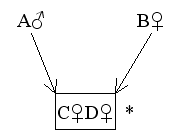Find RCD
 Common ancestors Paths (1/2)n None None (1/2)0 = 1.0 Therefore, RCD = Σ(1/2)n = 1.0

Why does (1/2)0 = 1.0 ?

Explanation (Following the normal rules of notation for power indices)

Since (1/2)1 = 1/2 and (1/2) -1 = 2/1 (i.e the reciprocal of 1/2)

Then by multiplication, 1/2 x 2 = 1.0 and (1/2)1 x (1/2) -1 = (1/2)1 - 1 = (1/2)0

Therefore, (1/2)0 = 1.0

__________________________________________________________________________________

* To indicate that C and D are identical twins, they are enclosed by a rectangular 'box'.

Identical Twin Common Ancestors

In tracing paths through ancestors who are identical twins, the twins are treated as though they are one person and therefore a single common ancestor. This is justified since they originated from a single fertilised egg (which can be regarded as the true common ancestor) and so their combined genetic contribution to the next generation is equivalent to that of a single individual.

Figure 14 Identical Twin Common Ancestors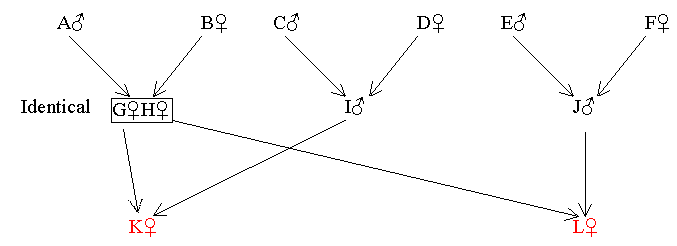Find RKL

Common Ancestors

Path

(1/2)n

 GH

 K_1_ GH _2_L

(1/2)2

=

1/4

Therefore RKL = 1/4 = 0.25

Table 1 Values of (1/2)n

 (1/2)0 1 1.0 (identical twins) (1/2)7 1/128 0.007 812 5 (1/2)1 1/2 0.5 (1/2)8 1/256 0.003 906 25 (1/2)2 1/4 0.25 (1/2)9 1/512 0.001 953 125 (1/2)3 1/8 0.125 (1/2)10 1/1024 0.000 976 562 5 (1/2)4 1/16 0.062 5 (1/2)11 1/2048 0.000 488 281 25 (1/2)5 1/32 0.031 25 (1/2)12 1/4096 0.000 244 140 625 (1/2)6 1/64 0.015 625 (1/2)13 1/8192 0.000 122 070 312 5

 There are a few slight corrections for different levels of inbreeding. However, since these corrections are generally small, and inbreeding appears to be rare in most families, they can usually be ignored. For the full extended formulae with worked examples see here in the appendix.

[2a] A and B in Figure 9, are said to be double great-great-grandparents of M.

 Without inbreeding in the family RAM would only have been 1/16.

 C, D, E and F are not common ancestors because each only passes genes to one of the two cousins. Also that the path G-D-A-E-H is the same as H-E-A-D-G.

 C, D, E and F can not be included as common ancestors because in order to move from I to J through each of them you must pass through the same individuals (G or H) more than once. This is contrary to rule (b).